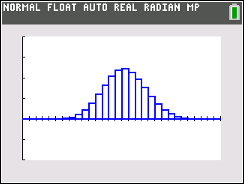### Mathematics lessons for IB® Diploma Programme

Applications and Interpretation | Statistics and Probability

# Binomial PDF Eye Color### Activity Overview

This activity involves binomial trials, distributions, and probabilities. Students can create the binomial distribution following the steps in Binomial_Pdf_Eye_Color_Create-84 document.

### Objectives

• Students will identify a situation involving binomial trials.
• Students will observe that a binomial distribution is a function of both sample
size and the probability of a success.
• Students will interpret a table of binomial probabilities.
• Student will answer probability questions using the graph of a binomial model for a
random variable involving binomial trials.

### Vocabulary

• binomial distribution
• expected value
• probability